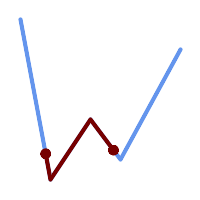## Name

ST_LineSubstring — Returns the part of a line between two fractional locations.

## Synopsis

`geometry ST_LineSubstring(`geometry a_linestring, float8 startfraction, float8 endfraction`)`;

`geography ST_LineSubstring(`geography a_linestring, float8 startfraction, float8 endfraction`)`;

## Description

Computes the line which is the section of the input line starting and ending at the given fractional locations. The first argument must be a LINESTRING. The second and third arguments are values in the range [0, 1] representing the start and end locations as fractions of line length. The Z and M values are interpolated for added endpoints if present.

If `startfraction` and `endfraction` have the same value this is equivalent to ST_LineInterpolatePoint.This only works with LINESTRINGs. To use on contiguous MULTILINESTRINGs first join them with ST_LineMerge.Since release 1.1.1 this function interpolates M and Z values. Prior releases set Z and M to unspecified values.

Enhanced: 3.4.0 - Support for geography was introduced.

Changed: 2.1.0. Up to 2.0.x this was called ST_Line_Substring.

Availability: 1.1.0, Z and M supported added in 1.1.1This function supports 3d and will not drop the z-index.

## ExamplesA LineString seen with 1/3 midrange overlaid (0.333, 0.666)

```SELECT ST_AsText(ST_LineSubstring( 'LINESTRING (20 180, 50 20, 90 80, 120 40, 180 150)', 0.333, 0.666));
------------------------------------------------------------------------------------------------
LINESTRING (45.17311810399485 45.74337011202746, 50 20, 90 80, 112.97593050157862 49.36542599789519)
```

If start and end locations are the same, the result is a POINT.

```SELECT ST_AsText(ST_LineSubstring( 'LINESTRING(25 50, 100 125, 150 190)', 0.333, 0.333));
------------------------------------------
POINT(69.2846934853974 94.2846934853974)
```

A query to cut a LineString into sections of length 100 or shorter. It uses `generate_series()` with a CROSS JOIN LATERAL to produce the equivalent of a FOR loop.

```WITH data(id, geom) AS (VALUES
( 'A', 'LINESTRING( 0 0, 200 0)'::geometry ),
( 'B', 'LINESTRING( 0 100, 350 100)'::geometry ),
( 'C', 'LINESTRING( 0 200, 50 200)'::geometry )
)
SELECT id, i,
ST_AsText( ST_LineSubstring( geom, startfrac, LEAST( endfrac, 1 )) ) AS geom
FROM (
SELECT id, geom, ST_Length(geom) len, 100 sublen FROM data
) AS d
CROSS JOIN LATERAL (
SELECT i, (sublen * i) / len AS startfrac,
(sublen * (i+1)) / len AS endfrac
FROM generate_series(0, floor( len / sublen )::integer ) AS t(i)
-- skip last i if line length is exact multiple of sublen
WHERE (sublen * i) / len <> 1.0
) AS d2;

id | i |            geom
----+---+-----------------------------
A  | 0 | LINESTRING(0 0,100 0)
A  | 1 | LINESTRING(100 0,200 0)
B  | 0 | LINESTRING(0 100,100 100)
B  | 1 | LINESTRING(100 100,200 100)
B  | 2 | LINESTRING(200 100,300 100)
B  | 3 | LINESTRING(300 100,350 100)
C  | 0 | LINESTRING(0 200,50 200)
```

Geography implementation measures along a spheroid, geometry along a line

```SELECT ST_AsText(ST_LineSubstring( 'LINESTRING(-118.2436 34.0522, -71.0570 42.3611)'::geography, 0.333, 0.666),6) AS geog_sub
, ST_AsText(ST_LineSubstring('LINESTRING(-118.2436 34.0522, -71.0570 42.3611)'::geometry, 0.333, 0.666),6) AS geom_sub;
---------------------------------------------------------------
geog_sub | LINESTRING(-104.167064 38.854691,-87.674646 41.849854)
geom_sub | LINESTRING(-102.530462 36.819064,-86.817324 39.585927)
```